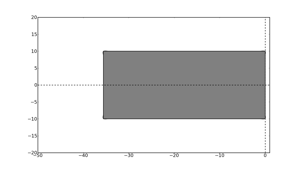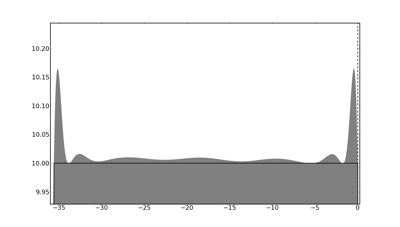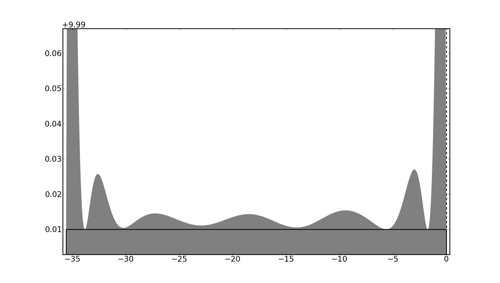# Can a region of absolute stability be rectangular?

When a one-step integrator is applied to the solution of the linear scalar ODE

$$u'(t) = \lambda u(t)$$

the resulting iteration takes the simple form

$$U^{n} = R(h\lambda) U^{n-1}$$

where $U^n$ is a numerical approximation to the solution $u(t_n)$ and $R(h\lambda)$ is called the stability function.  The details of the stability function depend on the choice of numerical method, but for any explicit Runge-Kutta method, $R(z)$ is a polynomial whose degree is at most the number of stages of the method.

The stability function completely characterizes the accuracy and stability of the method when applied to linear problems.  Consider the first order linear, autonomous ODE

$$u'(t) = L u(t)$$

where now $u$ is a vector and $L$ is a square matrix.  The numerical solution will be

$$U^{n} = R(hL) U^{n-1}.$$

The global error satisfies a similar recurrence; in particular, it gets multiplied by a factor $R(hL)$ at each step.  Let $\lambda$ denote any eigenvalue of $L$; then If $L$ is a normal matrix, the solution will be absolutely stable in the Euclidean norm if all values $h\lambda$ lie within the stability region $S$, defined as

$$S = \{ z\in\mathbb{C} : |R(z)|\le 1\}.$$

Thus the region of absolute stability defines the portion of the complex plane in which a given numerical integration method may appropriately be applied.

In our preprint on Runge-Kutta stability regions, Aron Ahmadia and I claim that we have an algorithm to generate a stability region appropriate for any spectrum.  By considering high-degree polynomials, we find that the resulting stability regions are tightly adapted to the shape of the imposed spectrum.

While this promises to be very useful for some problems, it also has an aspect that's just fun: we can generate stability regions with unusual shapes.  I haven't explored this much yet, but a first question that we ask in the preprint is how to generate a stability region for a spectrum of eigenvalues forming a rectangle in the left half of the complex plane.

Here is an example of a resulting stability region:The gray region is the set $S$ for a certain degree-20 stability polynomial corresponding to a consistent twenty-stage Runge-Kutta method.  As one colleague told me when I showed it to him, "this seems too good to be true; is that rectangle really the stability region?"

Indeed it is.  Zooming in on the top edge we see the detailed structure of the boundary:Zooming in even closer:As is typical with optimal stability polynomials, we se that the boundary is tangent or nearly tangent to the desired region at a large number of points (about 20 in this case).

What other shapes can be approximated?  More on that later...